# Navigating between oscilloscope-land (Volts) and RF-land (dBm)

Have you ever been annonyed by the dBm and V units when you try to measure output from your benchtop signal generator (or arbitrary waveform generator, or ARB) with a spectrum analyzer, or measure a RF signal (sine) generator with an oscilloscope?

## TLDR

For $50\Omega$ systems only. The fastest way is to work with squared voltages in linear scale with the provided ‘magic’ scaling factor (10 for amplitude and 20 for rms) and not mess with factorization in the log (dB) or anti-log ($10^{\frac{\mathrm{dB}}{10}}$) process:

P[\mathrm{mW}]=10V_{pp}^2=20V_{rms}^2
\mathrm{dBmW}=\mathrm{dB}\left(10V_{pp}^2\right)=\mathrm{dB}\left(20V_{rms}^2\right)

This happens a lot during calibration. An oscilloscope needs a 500Mhz signal at $600mV_{pp}$ to test if the front-end rolls off too early (i.e. have a lower bandwidth than advertised), yet my \$12k RF signal generator (20Ghz sweeper) only does dBm, and in coarse increments of 0.1dBm. • Decibel is supposed to be a dimensionless quantity, aka just a ratio. • The confusion came from people in each field abusing notations by skipping the reference quantity suffix after dB. e.g. RF people write dBW and dBmW (a dimensioned quantity with loaded assumptions of a$50\Omega$scaling) as dB and dBm (which would naturally read as a dimensionless ratio) • When RF people talk about decibels, especially when they abuse notations by not mentioning the reference (basis) quantity, you can be pretty sure that they are talking about ratios between power quantites (dBW), not voltage quantities (such as dBV). • There’s no such thing as a$20log_{10}$decibel unit. It’s always$10log_{10}$dimensionless. The factor of 2 came from the square (power of 2) factoring out of logarithms as a multiple of 2 when ratios of power are expressed as ratios of voltages. • Always work in power ratios to avoid the confusion. dB is dimensionless but often the unspoken rule is that it’s a ratio of power quantities. Routines or formula like mag2db really mean magnitude_squared_in_dB because it’s doing$10log_{10}(A^2)$. • The other advantage of actually squaring the term first before taking the log is that you do not have to check the input for negative values as the squaring operation made it absolute value (square). If you are doing a logarithm, an extra multiplication (over an addition) is insignicant in terms of computational burden. •$V_{rms}$maps directly to power quantities, scaled by the impedance (which is typically$50\Omega$). Everything else involves an extra crest factor (which is$\sqrt{2}\$ for sinusoids)# An easy way to remember Euclid GCD algorithm: laying tiles

I was a little embarrassed that I’ve never came across Euclid’s GCD algorithm until years after I graduated with Math and Engineering degrees. The descriptions I’ve found online (especially Wikipedia) are convoluted and confusing, and the mechanical description of the algorithm does not shine light to any intuition. Then there comes proofs and abstract algebra properties that even after I followed the reasoning, I’d soon forget after I stop looking at it in a few weeks.

Just by staring at one simple description of the algorithm:

The classic algorithm for computing the GCD, known as Euclid’s algorithm, goes as follows: Let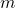and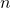be variables containing the two numbers, divideby. Save the divisor in, and save the remainder in. Ifis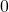, then stop:contains the GCD. Otherwise repeat the process, starting with division ofby.

K.N. King’s C++ Programming (Chapter 6 Exercise 2)

I reversed engineered one line of reasoning how one could come up with the Euclid GCD on his own. Turns out there is one twist/angle that most traditional explanations glossed over: quotients do NOT matter and WHY it does NOT matter!

It goes back to what GCD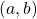stands for: greatest common divisor. You are looking for the largest factor that simultaneously divides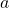and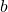evenly. If you rethink in terms of multiplication, you are finding the biggest tile size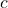that can simultaneously coverandwithout gaps:

Finds.t.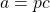and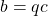where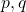are integers.

Imagine you are a lazy and cheap contractor who have unlimited tile samples of all sizes to try before making a large order:

• [Goal] you have to cover with two floors with potentially different sizesandgaplessly
• [Constraint: divisible] your customer won’t let you cut (fractional) tiles because it’s ugly
• [Constraint: common denominator] you can only order ONE tile size to take advantage of bulk discounts
• [Objective: greatest] more tiles means more work to lay it, so you want to shop for the biggest tile size that does the job.

For simplicity of the argument, we also assume the divisoris the smaller number. Ifis the bigger number, the roles will reverse in the next round because the remainder from dividing by a larger number is the dividend itself, which becomes the divisor in the next round while the old divisor (the larger number) becomes the dividend.

For relatively prime pairs, the algorithm will eventually stop with a GCD ofbecausedivides all integers.

Here’s the analog of Euclid algorithm:

• start out with 1 big tile covering the entire smaller floor• see if we can coverwithout gaps using big tiles of size• if yes, we are done., the macro-tile is your GCD (perfect-sized tile).
• if there are gaps (non-zero remainder), pick a tile-size that covers the ugly gap (the remainder becomes the divisor), then repeat the same process above over the last tile size(the divisor becomes the dividend) until there are no gaps (remainder become zero).

The algorithm is taking advantage of the fact the remainder is smaller than the last tile size (divisor) so we can rewrite the last tile size in multiples of the remainder (old gap) plus the new gap. By the time the new gap evenly divides the last tile (the big tile right before it), all numbers before that can be written as multiples of the new gap (i.e. the last gap evenly divides all the numbers in the chain all the way up toand).

Let’s start with a simple case GCD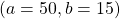that terminates early: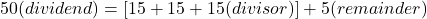We focus on the last tile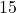and write it in terms of the old remainder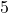: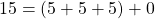Since the new remainder is, the old remainderdivides the last tile. Since we are dividingin terms of the remainder, we have

\begin{aligned}
b &= 15 \\
&= (5+5+5) \\
&= 3r \\
\\
a &= 50 \\
& = (15 + 15 + 15) + 5\\
& = 3b + r\\
&= (5+5+5) + (5+5+5) + (5+5+5) + 5\\
&= 3(3r)+r \\
& = 10r
\end{aligned}

So bothandcan be written in terms of integer multiples of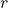right before the algorithm stops.

GCD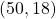takes a few more steps:

\begin{aligned}
b &= 18\\
a &= 50\\
&= (18 + 18) + 14\\
&=2b + r\\
r &= 14 \\
\\
b_1 &= r = 14\\
a_1 &= b = 18\\
&= (14) + 4\\
&= b_1 + r_1\\
r_1 &= 4\\
\\
b_2 &=r_1 = 4\\
a_2 &= b_1 = 14\\
&= (4+4+4)+2\\
&= 3b_2 + r_2\\
r_2 &= 2\\
\\
b_3 &= r_2 = 2\\
a_3 &= b_2 = 4\\
&=(2+2) + 0\\
r_3 &= 0\\

\end{aligned}

The algorithms stops atwhich means we successfully divided the last tile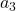with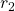(logistically stored in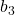) with no gaps left.(oris therefore the greatest common factor (divisor) that are shared by all the numbers involved all the way up toand.

This is the beauty of Euclid’s GCD algorithm: once the gap (remainder) is sliced small enough to evenly divide the tile (divisor) right before it, every term before it be can written in terms of integer multiples of the last number that divides the last tile without gap (no more remainder).

In our example,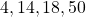can be written as a multiple of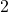, akabecause integer multiples of numbers that are already integer multiples ofcan be written in terms of a larger integer multiple of. This is why quotients do not matter in the Euclid GCD algorithm:

\begin{aligned}
b_2 &= 2r_2\\
4 &= (2+2) \\
\\
b_1 &= 3b_2 + r_2\\
&= 3(2r_2) + r_2\\
& = 7r_2\\
14 &= [(4+4+4)+2] \\
&=  [(2+2)+(2+2)+(2+2)+2] \\

\\
b &= b_1 + r_1\\
&=7r_2 + 2r_2\\
&=9r_2\\
18 &= 14 + 4 \\
&=  [(4+4+4)+2] + 4 \\
&=  [(2+2)+(2+2)+(2+2)+2]+[(2+2)] \\
\\
a &= 2b + r\\
&=2(9r_2) + 7r_2\\
&= 25r_2\\
50 & = 18 + 18 + 14 \\
&= [(2+2)+(2+2)+(2+2)+2]+[(2+2)] \cdots \\
&+ [(2+2)+(2+2)+(2+2)+2]+[(2+2)] \cdots \\
&+ [(2+2)+(2+2)+(2+2)+2]
\end {aligned}

The spirit of the algorithm is that every time we see a gap, we fill it with a small tile that’s exactly the gap size, and attempt to replace the last tile by covering it in terms of the new smaller tile (that was used to fill the gap). We are recursively slicing the last tile with the gaps produced until there are no gaps left. Once you find the GCD tile, you can replace all the bigger tiles before in terms of it with no gaps.

There’s an interesting perspective of using the Euclid GCD algorithm to solve Diophantine Equations (integer written as a linear combination of integers with integer coefficients): turns if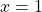in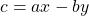is the same as writing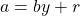. We can flip the sign of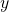by saying substituting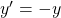.

In summary,

Euclid GCD’s algorithm is sub-dividing the last (or any one) large tile (last divisor) with the gap (last remainder) until there are no gaps left. Then any bigger tiles up the chain can be expressed as an integer multiple of the last piece (the smallest piece searched so far) that fills the last gap.

The first large tile is of course the smaller of the two numbers involved in the gcd calculation. The larger of the two number is the floor to be covered.

The other observation is that when you see the remainder being 1, you already know the two numbers are relatively prime. The last step to get the remainder to 0 is redundant (because it’s a guaranteed last step).

The other observation is that subdividing the last big tile (divisor) with the last gap (remainder) till it’s evenly divided chains up, because everything is an integer multiple of the smallest piece that evenly divides the gap (remainder) and the last big tile (divisor), therefore the algorithm is recursive.

\gcd(r_1,r_2) = \gcd(r_2, r_3) = ... = \gcd(r_{n-1}, r_n)

But this kind of recursion is not mandatory. It’s a tail recursion* that can be unrolled into iterations (looping), either by the compiler or the programmer, which is what we initially did.# HP 54502A Datasheet typo about AC coupling

The cutoff frequency of 10Hz on the datasheet is a typo. Better scopes at the time claims 90Hz. 10Hz is just too good to be true.

Found the specs from the service manual:

Don’t be fooled by the -3dB cutoff and ignore how wide the transition band can be (depends on the filter type and the order). Turns out this model has a very primitive filter that AC couple mode still messes square waves below 3kHz up despite the specs says the -3dB is at 90Hz. You better have a 30+ fold guard band for old scopes!

Remember square wave pulse train in time domain is basically a sinc pulse centered at every impulse of the impulse train in frequency domain superimposed. Unless you have a tiny duty cycle (which is not the case for uniform square waves, they are 50%), the left hand side of the sinc function at 1kHz fundamental still have sub-1kHz components that can be truncated by the AC coupling (high pass filter).# System Engineers’ tip to HKCEE/HKALE Math and Physics

Shortly after I’ve graduated with Mathematics and Electrical (and Computer) Engineering degrees, I realized a few supposedly difficult topics in Hong Kong’s Mathematics and Physics (Electric Circuits) curriculum was taught in unnecessarily painful ways.

Here’s an article I’ve written to show that it is less work to teach secondary (high) school students a few easy-to-learn university math topics first than teaching them dumb and clumsy derivations/approaches to avoid the pre-requisitesHKDSE EE Tips

Here are the outline of the article

• Complex numbers with Euler Formula
• Trigonometric identities can be derived effortlessly using complex number than tricky geometric proofs
• Inverting matrices using Gaussian elimination instead of messing with cofactors and determinants
• Proper concepts of circuit analysis and shortcuts
• Solving AC circuits in a breeze with complex numbers instead of remembering stupid rules like ELI and ICE rules and messy trigonometric identities.# 變幻才是永恆 = 世界是線性?

– Sep 17, 2005